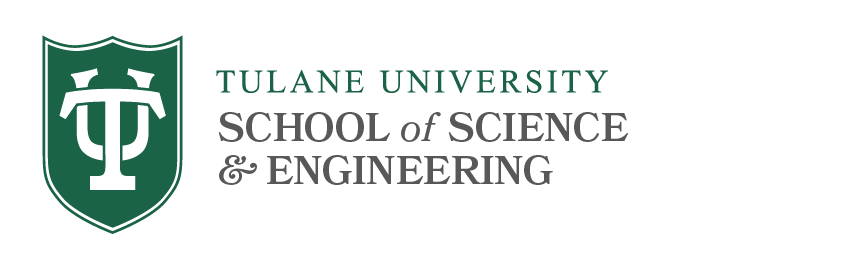# Analysis Qualifying Exams

## Syllabus Topics

This exam will test your working knowledge of basic real, complex and functional analysis. You will be required to demonstrate an ability to use standard results and techniques to solve problems, including special cases of standard theorems which do not require long arguments.

We will not emphasize the memorization of statements of theorems nor of long proofs of standard theorems.

The student is urged to work on the problems in the relevant sections and chapters in the reference books.

Syllabus (updated Fall 2020).
There will typically be 12 problems, 7 from real analysis and 5 from complex analysis. Students must designate 10 to be graded.

### Real Analysis

• Abstract measure spaces and construction of measures.
• Measurable functions and general theory of integration.
• Integral Convergence Theorems (Fatou, Monotone, Dominated, Vitali).
• Modes of convergence: convergence a.e.; convergence in measure and convergence in the mean and how they are related to each other, Egorov's Theorem, Luzin's Theorem.
• Absolute continuity and the Radon-Nikodym Theorem.
• Product Measures and Fubini-Tonelli Theorem
• Lebesgue Differentiation Theorem; Comparison of Riemann and Lebesgue integrals; Bounded Variation etc.
• Lp spaces with 1≤p≤∞, Hölder inequality, Minkowski inequality, Jensen's inequality, convergence in Lp, completeness of spaces, dense sets of Lp( a,b) (1≤p<∞).

References
  Natanson, Theory of Functions of a Real Variable.
  Kolmogorov and Fomin, Introductory Real Analysis.
  Royden, Real Analysis.
  Rudin, Real and Complex Analysis, 2nd edition.
  Friedman, Foundations of Modern Analysis.
  Folland, Real Analysis.
  Wheeden and Zygmund, Measure and Integral, an introduction to real analysis.
  Stein and Sharkarchi, Real Analysis, Measure Theory, Integration and Hilbert Spaces.
  Stein and Sharkarchi, Fourier Analysis.

### Complex Analysis

• Definition of Holomorphic functions with examples, including logarithms, roots, and Möbius transformations.
• Cauchy-Riemann Equations.
• Power Series Expansion and applications including the Identity Theorem.
• Cauchy Integral Theorem.
• Reimann Mapping Theorem
• Applications of Cauchy Integral Theorem to evaluating Riemann integrals and summation of infinite series, Rouché's Theorem, The Argument Principle, Open Mapping Theorem, Liouville's Theorem, The Fundamental Theorem of Algebra.

References
  Ahlfors,Complex Analysis
  Conway, Functions of One Complex Variable
  Schaum's Outline of Complex Variables